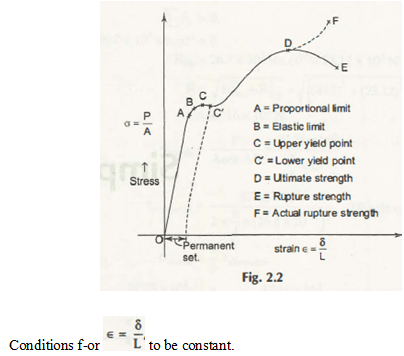Stress is P/A and Strain is δ/L.

Here, c is considered is constant.

For the mild steel, and for the semi ductile there are some important factors important as –

• The suspended load is axial and thus it must generate a uniform stress.
• The homogeneous material is required.
• Cross-section of the specimen must be constant.

In the above diagram, you can see a number of fundamental concepts related to the curve and it is very important to understand each factor –

• Proportional Limit (A) –

A is the exact point in the diagram where stress ∝ strain.

• Elastic Limit (B) –

In the diagram of stress – strain, B is the elastic limit that indicates that elastic material regains its exact position like before without fixing up any permanent set.

• Yield Point as C or C’

Without increasing of stress there is an appreciable deformation. In this diagram, you can see two different points as C and C’ and they are known as yield points.

• Ultimate Stress (D)

Stress –Strain diagram this is the highest Ordinate.

• Rupture Stress (E)

At the point where the material or the object breaks is known rupture stress.  Calculation of the stress is very important for a proper and nominal diagram. Moreover, it is also important for you to understand that E will be lower than the value of ultimate stress (D).

• Actual Rupture Stress (F)

It can easily be calculated on the basis of the actual area and this can be taken as the narrow down or the necking phenomena.

Links of Next Mechanical Engineering Topics:-### Customer Reviews

My Homework Help
Rated 5.0 out of 5 based on 510 customer reviews at